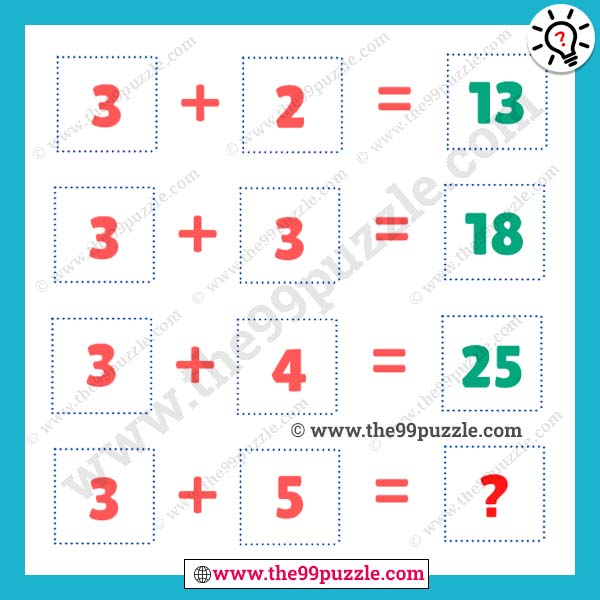# Logic of mathematics puzzle for teens with answer – Puzz291

Logical riddles and viral math equations can only solve the genius people. In this logic of mathematics puzzle, you will see some math equations. So, now you have to find out the logic and solve the problem. This type of logical math problem is very challenging and interesting. If you understand the logic you can easily find out the value of the last equation. These are mind-blowing questions for genius people. The puzzle makes you smarter and more genius. Learn more math tricks and shortcut math to solve these math puzzles.

3+2=13

3+3=18

3+4=25

3+5=?###### Explanation:

3+2 = 3²+2² = 9+4 = 13

3+3 = 3²+3² = 9+9 = 18

3+4 = 3²+4² = 9+16 = 25

3+5 = 3²+5² = 9+25 = 34